# Blood relation for IBPS, SBI, RBI, RRBs: Quiz 8

## Blood relation for IBPS, SBI, RBI, RRBs: Quiz 8

We are providing you with the Blood Relation Quiz here. Blood relation cover 3-4 questions in the reasoning section in government exams, and we are providing detailed explanations which will help you develop a simple approach to answer Blood relation questions.

The Blood Relation Questions that we are providing are useful for SSC, Railways, Banking & Insurance etc.

Practicing this Blood relation quiz will make this topic easier.

This Blood Relation Quiz will help in upcoming SSC, Railways, and bank examinations.

Directions (1-2): Study the following information carefully and answer the questions which follow–

‘M × N’ means ‘M is wife of N’

‘M ¸ N’ means ‘M is husband of N’

‘M – N’ means ‘M is father of N’

‘M + N’ means ‘M is sister of N’

1. If expression ‘A + B – D + G × Q’ is true then which of the following is true?
(a) A is mother-in-law of Q

(b) Q is daughter-in-law of B

(c) G is nephew of A

(d) B is father of G

(e) None of these1. Which of the following means ‘D is mother-in-law of M’
(a) D × V – A ¸ R + M – T

(b) D + A – V × T – R ¸ M

(c) D × T – V + R ¸ M

(d)  R ¸ D + T × A – V + M

(d) None of these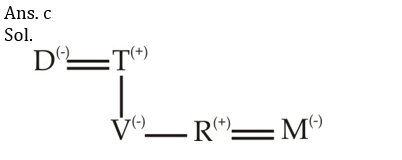1. Study the following information carefully and answer the questions which follow–

Y = Z’ means ‘Y is wife of Z’

‘Y – Z’ means ‘Y is daughter of Z’

‘Y ¸ Z’ means ‘Y is father of Z’

‘Y × Z’ means ‘Y is mother of Z’

‘Y + Z’ means ‘Y is son of Z’

If expression ‘B + M = C ¸ G × E – D’ is true then how is D related to B?
(a) father

(b) father-in-law

(c) brother

(d) nephew

(e) brother-in-lawDirections (4-6) : Study the following information carefully and answer the questions given below:

In a three-generation family, there are seven members Rina, Ashu, Rupa, Julie, Vishak, Roshini and Keshav, in which there are two married couples. Rina is sister of Ashu, who is maternal grandson of Vishak. The father of Julie has two maternal grandchildren. Rupa is sister-in-law of Keshav, who is father of Rina. Roshini is a female and Keshav has no brothers.

1. What is the relation between Julie and Roshini?

(a) Roshini is mother of Julie

(b) Julie is brother of Roshini

(c) Julie is son of Roshini

(d) Julie is daughter-in-law of Roshini

(e) Can’t be determined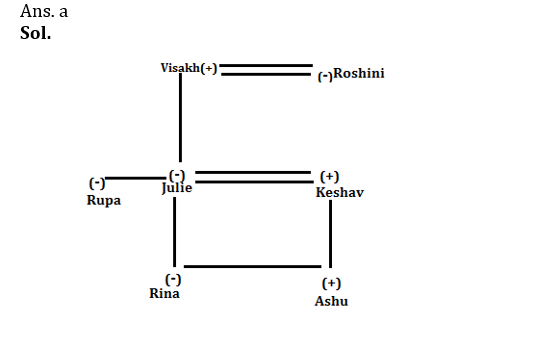1. Who among the following is husband of Roshini?

(a) Keshav

(b) Juilie

(c) Rina

(d) Vishak

(e) None of these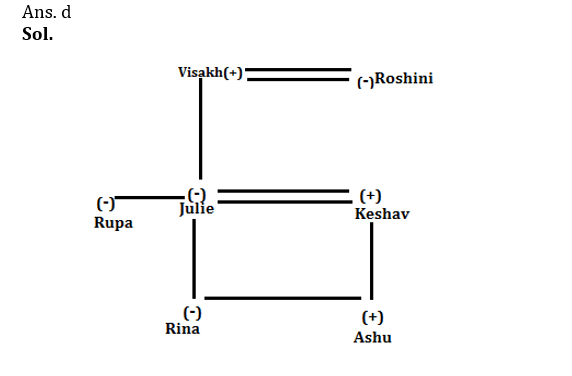1. How Rina is related to Julie?

(a) Rina is brother of Julie

(b) Rina is daughter of Julie

(c) Rina is sister of Julie

(d) Rina is father of Julie

(e) None of these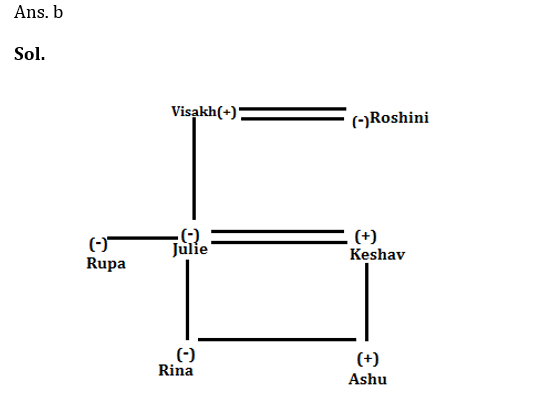Directions (7-10): Study the following information carefully and answer the questions which follow–

‘K @ R’ means ‘K is wife of R’

‘K # R’ means ‘K is son of R’

‘K \$ R’ means ‘K is father of R’

‘K % R’ means ‘K is sister of R’

1. Which of the following expression represents the relationship ‘T is mother of N’?

(a) T @ R \$ P % N

(b) T @ R # P \$ N

(c) T % R \$ P @ N

(d) T \$ R % P \$ N

(e) T # R % P @ N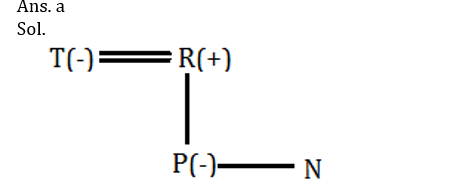1. In expressions ‘A % B # C \$ D’ how is B related to D?

(a) brother-in-law

(b) sister

(c) brother

(d) cousin

(e) None of these1. Which of the following expression represents the relationship ‘M is brother of G’?

(a) G # T \$ M

(b) G % T \$ M

(c) M % G \$ T

(d) G % M \$ T

(e) M # T % G1. In expressions ‘A % B # C \$ D’ how is A related to D?

(a) brother-in-law

(b) sister

(c) brother

(d) cousin

(e) None of these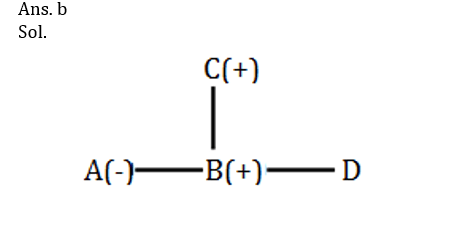#### Aatma Nirbhar Reasoning Topic Wise Online Test Series

Recommended PDF’s for 2021:

### Preparation Kit PDF

#### Most important PDF’s for Bank, SSC, Railway and Other Government Exam : Download PDF Now

AATMA-NIRBHAR Series- Static GK/Awareness Practice Ebook PDF Get PDF here
The Banking Awareness 500 MCQs E-book| Bilingual (Hindi + English) Get PDF here
AATMA-NIRBHAR Series- Banking Awareness Practice Ebook PDF Get PDF here
Computer Awareness Capsule 2.O Get PDF here
AATMA-NIRBHAR Series Quantitative Aptitude Topic-Wise PDF 2020 Get PDF here
Memory Based Puzzle E-book | 2016-19 Exams Covered Get PDF here
Caselet Data Interpretation 200 Questions Get PDF here
Puzzle & Seating Arrangement E-Book for BANK PO MAINS (Vol-1) Get PDF here
ARITHMETIC DATA INTERPRETATION 2.O E-book Get PDF here
3